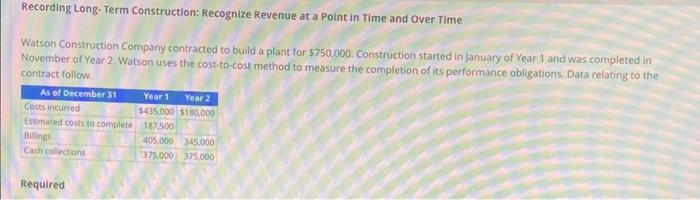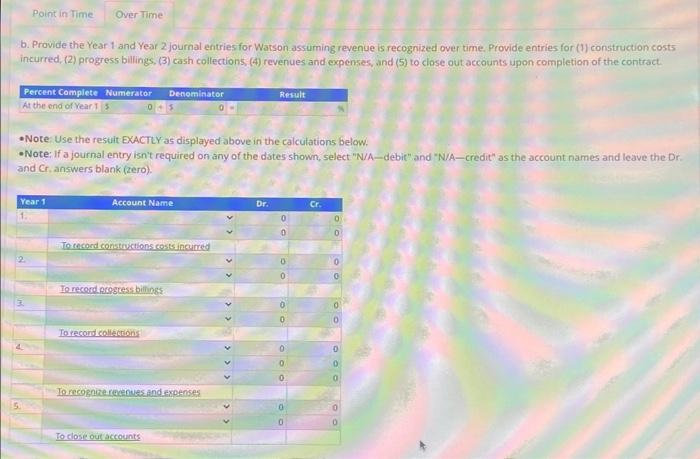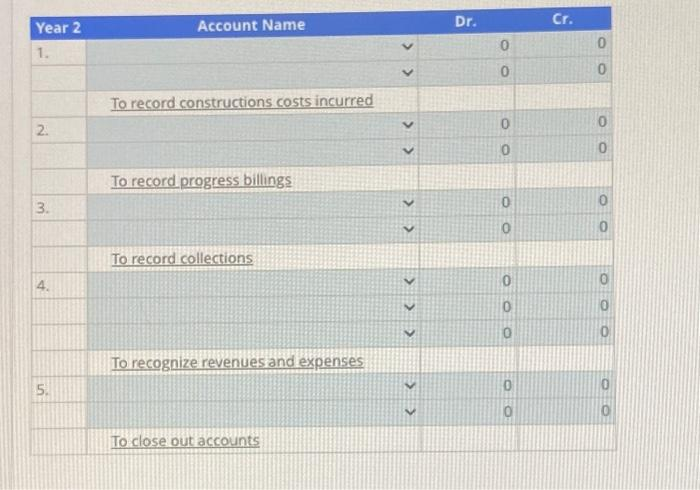# Question Recording Long. Term Construction: Recognize Revenue at a Point in Time and Over Time Watson Construction Company contracted to build a plant for $$\ 750,000$$. Construction started in january of Year 1 and was completed in November of Year 2. Watson uses the cost-to-cost method to measure the completion of its performance obligations. Data relating to the contract follow. Required b. Provide the Year 1 and Year 2 journal entries for Watson assuming revenue is recognized over time. Provide entries for (1) construction costs incurred, (2) progress billings, (3) cash collections, (4) revenues and expenses, and (5) to close out accounts upon completion of the contract. - Note: Use the restit EXACTLY as displayed above in the calculations below. - Note: If a journal entry isn't required on any of the dates shown, select "N/A-debie" and "N/A-credit" as the account names and leave the Dr. and $$\mathrm{Cr}$$, answers blank (zero). To record collections 4. 5. To recognize revenues and expenses To close out accountsTranscribed Image Text: Recording Long. Term Construction: Recognize Revenue at a Point in Time and Over Time Watson Construction Company contracted to build a plant for $$\ 750,000$$. Construction started in january of Year 1 and was completed in November of Year 2. Watson uses the cost-to-cost method to measure the completion of its performance obligations. Data relating to the contract follow. Required b. Provide the Year 1 and Year 2 journal entries for Watson assuming revenue is recognized over time. Provide entries for (1) construction costs incurred, (2) progress billings, (3) cash collections, (4) revenues and expenses, and (5) to close out accounts upon completion of the contract. - Note: Use the restit EXACTLY as displayed above in the calculations below. - Note: If a journal entry isn't required on any of the dates shown, select "N/A-debie" and "N/A-credit" as the account names and leave the Dr. and $$\mathrm{Cr}$$, answers blank (zero). To record collections 4. 5. To recognize revenues and expenses To close out accounts
Transcribed Image Text: Recording Long. Term Construction: Recognize Revenue at a Point in Time and Over Time Watson Construction Company contracted to build a plant for $$\ 750,000$$. Construction started in january of Year 1 and was completed in November of Year 2. Watson uses the cost-to-cost method to measure the completion of its performance obligations. Data relating to the contract follow. Required b. Provide the Year 1 and Year 2 journal entries for Watson assuming revenue is recognized over time. Provide entries for (1) construction costs incurred, (2) progress billings, (3) cash collections, (4) revenues and expenses, and (5) to close out accounts upon completion of the contract. - Note: Use the restit EXACTLY as displayed above in the calculations below. - Note: If a journal entry isn't required on any of the dates shown, select "N/A-debie" and "N/A-credit" as the account names and leave the Dr. and $$\mathrm{Cr}$$, answers blank (zero). To record collections 4. 5. To recognize revenues and expenses To close out accounts&#12304;General guidance&#12305;The answer provided below has been developed in a clear step by step manner.Step1/3Accounting for Construction Contracts- Percentage of Completion methodYear 1:Cost incurred to date = $435,000Estimated future costs to complete =$187,500\begin{align*} \mathrm{Total\space estimated\space costs\space of\space contract} & = \mathrm{cost\space incurred\space to\space date+estimated\space future\space costs\space to\space complete} \\& = \mathrm{\{435{,}000}+{187{,}500}} \\& = \mathrm{\{622}{,}{500}} \end{align*}Numerator for percent complete = Cost incurred to dateDenominator for percent complete = Total estimated costs of contract\begin{align*} \mathrm{Percent\space complete} & = \mathrm{cost\space incurred\space to\space date/total\space estimated\space costs*100} \\& = \mathrm{\frac{{435{,}000}}{{622{,}500}}\times{100}} \\& = \mathrm{{69.88}\%} \end{align*}(Here, the percent complete is taken with two decimal places by default. It can also be taken with lesser or no decimal places if the question specifically states. So please use the appropriate decimal places as guided by the question.)Explanation:Under the percentage of completion method, the revenue from the contract in progress is recognized based on the percentage completed. The percent complete is determined using the costs already incurred to date and the total estimated cost of the whole contract. This percentage is used to calculate the construction revenue to be recognized for the year. Step2/3Accounting for Construction Contracts- Percentage of Completion methodYear 1:Percent complete = 69.88% or 70%Cost incurred to date = $435,000Total contract price =$750,000\( \begin{align*} \mathrm{Contract\space revenue\space to\space recognize\space in\space yea ... See the full answer